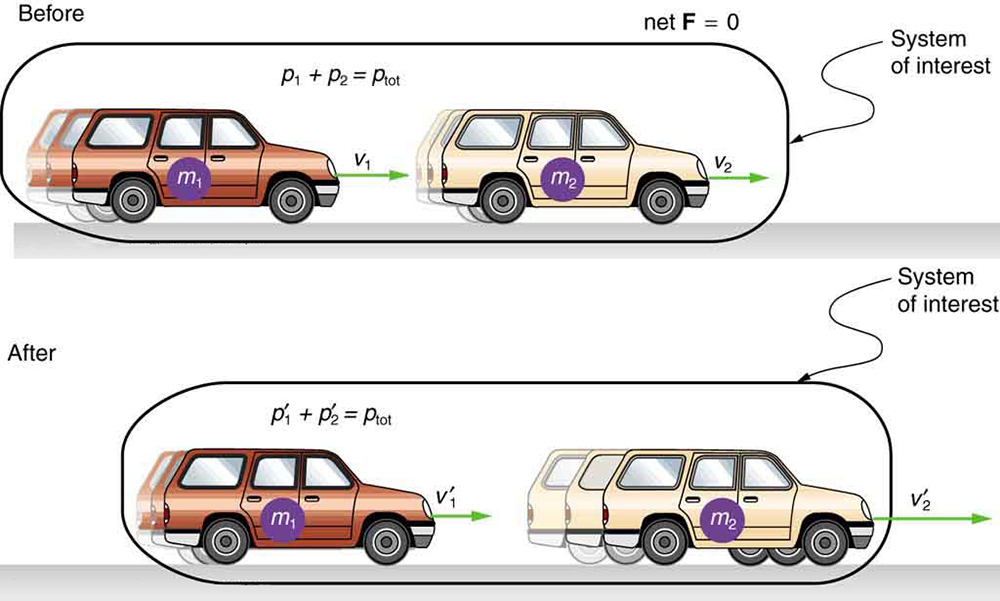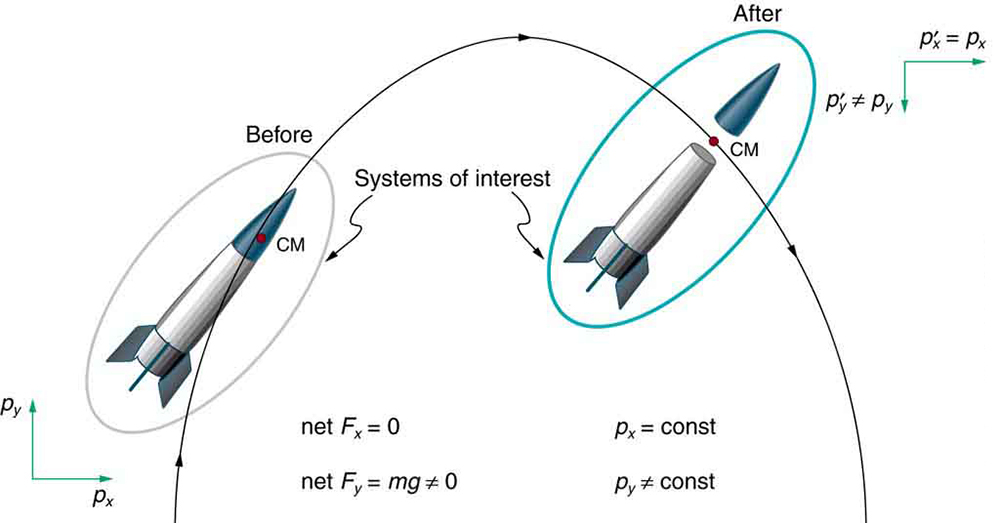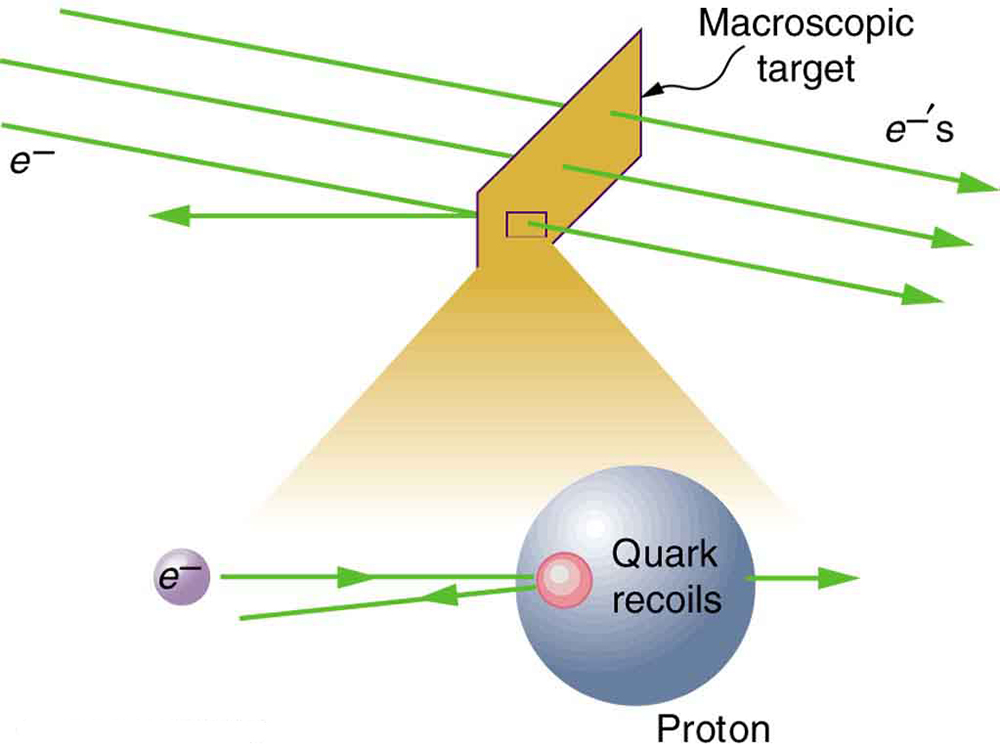Chapter 8 Linear Momentum and Collisions

# 8.3 Conservation of Momentum

### Summary

• Describe the principle of conservation of momentum.
• Derive an expression for the conservation of momentum.
• Explain conservation of momentum with examples.
• Explain the principle of conservation of momentum as it relates to atomic and subatomic particles.

Momentum is an important quantity because it is conserved. Yet it was not conserved in the examples in Chapter 8.2 Impulse and Chapter 8.1 Linear Momentum and Force, where large changes in momentum were produced by forces acting on the system of interest. Under what circumstances is momentum conserved?

The answer to this question entails considering a sufficiently large system. It is always possible to find a larger system in which total momentum is constant, even if momentum changes for components of the system. If a football player runs into the goalpost in the end zone, there will be a force on him that causes him to bounce backward. However, the Earth also recoils —conserving momentum—because of the force applied to it through the goalpost. Because Earth is many orders of magnitude more massive than the player, its recoil is immeasurably small and can be neglected in any practical sense, but it is real nevertheless.

Consider what happens if the masses of two colliding objects are more similar than the masses of a football player and Earth—for example, one car bumping into another, as shown in Figure 1. Both cars are coasting in the same direction when the lead car (labeled$\boldsymbol{m_2}$) is bumped by the trailing car (labeled$\boldsymbol{m_1}$). The only unbalanced force on each car is the force of the collision. (Assume that the effects due to friction are negligible.) Car 1 slows down as a result of the collision, losing some momentum, while car 2 speeds up and gains some momentum. We shall now show that the total momentum of the two-car system remains constant.Figure 1. A car of mass m1 moving with a velocity of v1 bumps into another car of mass m2and velocity v2 that it is following. As a result, the first car slows down to a velocity of v‘1and the second speeds up to a velocity of v‘2. The momentum of each car is changed, but the total momentum ptotof the two cars is the same before and after the collision (if you assume friction is negligible).

Using the definition of impulse, the change in momentum of car 1 is given by

$\boldsymbol{\Delta{p}_1=F_1\Delta{t},}$

where$\boldsymbol{F_1}$is the force on car 1 due to car 2, and$\boldsymbol{\Delta{t}}$is the time the force acts (the duration of the collision). Intuitively, it seems obvious that the collision time is the same for both cars, but it is only true for objects traveling at ordinary speeds. This assumption must be modified for objects travelling near the speed of light, without affecting the result that momentum is conserved.

Similarly, the change in momentum of car 2 is

$\boldsymbol{\Delta{p}_2=F_2\Delta{t},}$

where$\boldsymbol{F_2}$is the force on car 2 due to car 1, and we assume the duration of the collision$\boldsymbol{\Delta{t}}$is the same for both cars. We know from Newton’s third law that$\boldsymbol{F_2=-F_1},$and so

$\boldsymbol{\Delta{p}_2=-F_1\Delta{t}=-\Delta{p}_1.}$

Thus, the changes in momentum are equal and opposite, and

$\boldsymbol{\Delta{p}_1+\Delta{p}_2=0.}$

Because the changes in momentum add to zero, the total momentum of the two-car system is constant. That is,

$\begin{array}{l} \boldsymbol{p_1+p_2=\textbf{constant},} \\ \boldsymbol{p_1+p_2=p^{\prime}_1+p^{\prime}_2,} \end{array}$

where$\boldsymbol{p^{\prime}_1}$and$\boldsymbol{p^{\prime}_2}$are the momenta of cars 1 and 2 after the collision. (We often use primes to denote the final state.)

This result—that momentum is conserved—has validity far beyond the preceding one-dimensional case. It can be similarly shown that total momentum is conserved for any isolated system, with any number of objects in it. In equation form, the conservation of momentum principle for an isolated system is written

$\boldsymbol{\textbf{p}_{\textbf{tot}}=\textbf{constant},}$

or

$\boldsymbol{\textbf{p}_{\textbf{tot}}=p^{\prime}_{\textbf{tot}},}$

where$\textbf{p}_{\textbf{tot}}$ is the total momentum (the sum of the momenta of the individual objects in the system) and$\boldsymbol{\textbf{p}^{\prime}_{\textbf{tot}}}$is the total momentum some time later. (The total momentum can be shown to be the momentum of the center of mass of the system.) An isolated system is defined to be one for which the net external force is zero$\boldsymbol{(\textbf{F}_{\textbf{net}}=0).}$

### CONSERVATION OF MOMENTUM PRINCIPLE

$\begin{array}{l} \boldsymbol{\textbf{p}_{\textbf{tot}}=\textbf{ constant}} \\ \boldsymbol{\textbf{p}_{\textbf{tot}}=\textbf{p}^{\prime}_{\textbf{tot}}\textbf{ (isolated system)}} \end{array}$

### ISOLATED SYSTEM

An isolated system is defined to be one for which the net external force is zero$\boldsymbol{(\textbf{F}_{\textbf{net}}=0).}$

Perhaps an easier way to see that momentum is conserved for an isolated system is to consider Newton’s second law in terms of momentum,$\boldsymbol{\textbf{F}_{\textbf{net}}=\frac{\Delta\textbf{p}_{\textbf{tot}}}{\Delta{t}}}.$For an isolated system,$\boldsymbol{\textbf{F}_{\textbf{net}}=0};$thus,$\boldsymbol{\Delta\textbf{p}_{\textbf{tot}}=0},$and$\textbf{p}_{\textbf{tot}}$is constant.

We have noted that the three length dimensions in nature—$\boldsymbol{x},\:\boldsymbol{y},$and$\boldsymbol{z}$—are independent, and it is interesting to note that momentum can be conserved in different ways along each dimension. For example, during projectile motion and where air resistance is negligible, momentum is conserved in the horizontal direction because horizontal forces are zero and momentum is unchanged. But along the vertical direction, the net vertical force is not zero and the momentum of the projectile is not conserved. (See Figure 2.) However, if the momentum of the projectile-Earth system is considered in the vertical direction, we find that the total momentum is conserved.Figure 2. The horizontal component of a projectile’s momentum is conserved if air resistance is negligible, even in this case where a space probe separates. The forces causing the separation are internal to the system, so that the net external horizontal force Fx-net is still zero. The vertical component of the momentum is not conserved, because the net vertical force Fy-net is not zero. In the vertical direction, the space probe-Earth system needs to be considered and we find that the total momentum is conserved. The center of mass of the space probe takes the same path it would if the separation did not occur.

The conservation of momentum principle can be applied to systems as different as a comet striking Earth and a gas containing huge numbers of atoms and molecules. Conservation of momentum is violated only when the net external force is not zero. But another larger system can always be considered in which momentum is conserved by simply including the source of the external force. For example, in the collision of two cars considered above, the two-car system conserves momentum while each one-car system does not.

### MAKING CONNECTIONS: TAKE-HOME INVESTIGATION—DROP OF TENNIS BALL AND A BASEBALL

Hold a tennis ball side by side and in contact with a basketball. Drop the balls together. (Be careful!) What happens? Explain your observations. Now hold the tennis ball above and in contact with the basketball. What happened? Explain your observations. What do you think will happen if the basketball ball is held above and in contact with the tennis ball?

### MAKING CONNECTIONS: TAKE-HOME INVESTIGATION—TWO TENNIS BALLS IN A BALLISTIC TRAJECTORY

Tie two tennis balls together with a string about a foot long. Hold one ball and let the other hang down and throw it in a ballistic trajectory. Explain your observations. Now mark the center of the string with bright ink or attach a brightly colored sticker to it and throw again. What happened? Explain your observations.

Some aquatic animals such as jellyfish move around based on the principles of conservation of momentum. A jellyfish fills its umbrella section with water and then pushes the water out resulting in motion in the opposite direction to that of the jet of water. Squids propel themselves in a similar manner but, in contrast with jellyfish, are able to control the direction in which they move by aiming their nozzle forward or backward. Typical squids can move at speeds of 8 to 12 km/h.

The ballistocardiograph (BCG) was a diagnostic tool used in the second half of the 20th century to study the strength of the heart. About once a second, your heart beats, forcing blood into the aorta. A force in the opposite direction is exerted on the rest of your body (recall Newton’s third law). A ballistocardiograph is a device that can measure this reaction force. This measurement is done by using a sensor (resting on the person) or by using a moving table suspended from the ceiling. This technique can gather information on the strength of the heart beat and the volume of blood passing from the heart. However, the electrocardiogram (ECG or EKG) and the echocardiogram (cardiac ECHO or ECHO; a technique that uses ultrasound to see an image of the heart) are more widely used in the practice of cardiology.

### MAKING CONNECTIONS: CONSERVATION OF MOMENTUM AND COLLISION

Conservation of momentum is quite useful in describing collisions. Momentum is crucial to our understanding of atomic and subatomic particles because much of what we know about these particles comes from collision experiments.

# Subatomic Collisions and Momentum

The conservation of momentum principle not only applies to the macroscopic objects, it is also essential to our explorations of atomic and subatomic particles. Giant machines hurl subatomic particles at one another, and researchers evaluate the results by assuming conservation of momentum (among other things).

On the small scale, we find that particles and their properties are invisible to the naked eye but can be measured with our instruments, and models of these subatomic particles can be constructed to describe the results. Momentum is found to be a property of all subatomic particles including massless particles such as photons that compose light. Momentum being a property of particles hints that momentum may have an identity beyond the description of an object’s mass multiplied by the object’s velocity. Indeed, momentum relates to wave properties and plays a fundamental role in what measurements are taken and how we take these measurements. Furthermore, we find that the conservation of momentum principle is valid when considering systems of particles. We use this principle to analyze the masses and other properties of previously undetected particles, such as the nucleus of an atom and the existence of quarks that make up particles of nuclei. Figure 3 below illustrates how a particle scattering backward from another implies that its target is massive and dense. Experiments seeking evidence that quarks make up protons (one type of particle that makes up nuclei) scattered high-energy electrons off of protons (nuclei of hydrogen atoms). Electrons occasionally scattered straight backward in a manner that implied a very small and very dense particle makes up the proton—this observation is considered nearly direct evidence of quarks. The analysis was based partly on the same conservation of momentum principle that works so well on the large scale.Figure 3. A subatomic particle scatters straight backward from a target particle. In experiments seeking evidence for quarks, electrons were observed to occasionally scatter straight backward from a proton.

# Section Summary

• The conservation of momentum principle is written
$\boldsymbol{p_{\textbf{tot}}=\textbf{constant}}$

or

$\boldsymbol{\textbf{p}_{\textbf{tot}}=\textbf{p}^{\prime}_{\textbf{tot}}\textbf{ (isolated system)},}$

$\textbf{p}_{\textbf{tot}}$is the initial total momentum and$\boldsymbol{\textbf{p}^{\prime}_{\textbf{tot}}}$is the total momentum some time later.

• An isolated system is defined to be one for which the net external force is zero$\boldsymbol{(\textbf{F}_{\textbf{net}}=0)}.$
• During projectile motion and where air resistance is negligible, momentum is conserved in the horizontal direction because horizontal forces are zero.
• Conservation of momentum applies only when the net external force is zero.
• The conservation of momentum principle is valid when considering systems of particles.

### Conceptual Questions

1: Professional Application

If you dive into water, you reach greater depths than if you do a belly flop. Explain this difference in depth using the concept of conservation of energy. Explain this difference in depth using what you have learned in this chapter.

2: Under what circumstances is momentum conserved?

3: Can momentum be conserved for a system if there are external forces acting on the system? If so, under what conditions? If not, why not?

4: Momentum for a system can be conserved in one direction while not being conserved in another. What is the angle between the directions? Give an example.

5: Professional Application

Explain in terms of momentum and Newton’s laws how a car’s air resistance is due in part to the fact that it pushes air in its direction of motion.

6: Can objects in a system have momentum while the momentum of the system is zero? Explain your answer.

7: Must the total energy of a system be conserved whenever its momentum is conserved? Explain why or why not.

### Problems & Exercises

1: Professional Application

Train cars are coupled together by being bumped into one another. Suppose two loaded train cars are moving toward one another, the first having a mass of 150,000 kg and a velocity of 0.300 m/s, and the second having a mass of 110,000 kg and a velocity of$\boldsymbol{-0.120\textbf{ m/s}}.$(The minus indicates direction of motion.) What is their final velocity?

2: Suppose a clay model of a koala bear has a mass of 0.200 kg and slides on ice at a speed of 0.750 m/s. It runs into another clay model, which is initially motionless and has a mass of 0.350 kg. Both being soft clay, they naturally stick together. What is their final velocity?

3: Professional Application

Consider the following question: A car moving at 10 m/s crashes into a tree and stops in 0.26 s. Calculate the force the seatbelt exerts on a passenger in the car to bring him to a halt. The mass of the passenger is 70 kg. Would the answer to this question be different if the car with the 70-kg passenger had collided with a car that has a mass equal to and is traveling in the opposite direction and at the same speed? Explain your answer.

4: What is the velocity of a 900-kg car initially moving at 30.0 m/s, just after it hits a 150-kg deer initially running at 12.0 m/s in the same direction? Assume the deer remains on the car.

5: A 1.80-kg falcon catches a 0.650-kg dove from behind in midair. What is their velocity after impact if the falcon’s velocity is initially 28.0 m/s and the dove’s velocity is 7.00 m/s in the same direction?

## Glossary

conservation of momentum principle
when the net external force is zero, the total momentum of the system is conserved or constant
isolated system
a system in which the net external force is zero
quark
fundamental constituent of matter and an elementary particle

### Exercises

Problems & Exercises

1:

0.122 m/s

3:

In a collision with an identical car, momentum is conserved. Afterwards$\boldsymbol{v_{\textbf{f}}=0}$for both cars. The change in momentum will be the same as in the crash with the tree. However, the force on the body is not determined since the time is not known. A padded stop will reduce injurious force on body.

5:

22.4 m/s in the same direction as the original motion